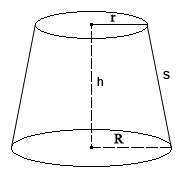# Conical Frustum Calculator

A frustum can be defined as a three-dimensional geometric figure that has its top removed by a plane parallel to the base.

The diameters of the top side and the base are parallel to each other and are located in the center of the axis.

It is important to recall some basic information that is relevant to the Frustum Calculator. The dimensions that can be found using the calculator include the vertical height of the frustum, the slant height of the sides, top and base radii.

Square units are used for the following dimensions: top surface area, lateral surface area, base surface area, and total surface area. The volume is calculated in cubic units.

Frustum Shape CalculatorYou may set the number of decimal places in the online calculator. By default there are only two decimal places.

Results
Base Surface Area: 0.00 sq. units
Top Surface Area: 0.00 sq. units
Lateral Surface Area: 0.00 sq. units
Total Surface Area: 0.00 sq. units
Volume: 0.00 cu. units

### The Conical Frustum Calculator is capable of applying the following algorithms:

Slant Height (s) = √((R − r)2+h2)

Base Surface Area = πR2

Top Surface Area = πr2

Lateral Surface Area = π * (r + R) * s

Total Surface Area = Base Surface Area + Top Surface Area + Lateral Surface Area

Volume = (1/3) * π * h * (r2 + R2 + (r * R))

Where π is the constant (3.141592654)